Practice Graphing Linear Equations Terms of Use    Contact Person: Donna RobertsDirections: These questions pertain to slopes and graphs of lines. Choose the correct answer.

1.
Find the slope (m) and y-intercept (b) for
y = 4x - 8.Choose:
 m = 4, b = 8 m = -4, b = 8 m = 4, b = -8 m = -4, b = -8

2.
What is the slope of the line shown in the graph at the right?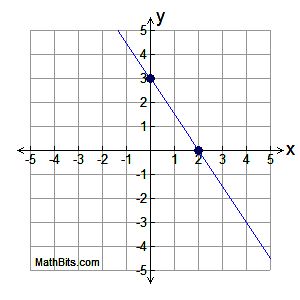Choose:
 m =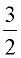m =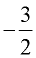m =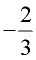m =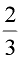3.
What is the equation of the line shown in the graph at the right?Choose: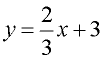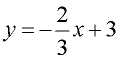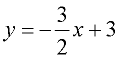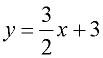4.
What is the slope of the line passing
through the points (0,-1) and (2,3)?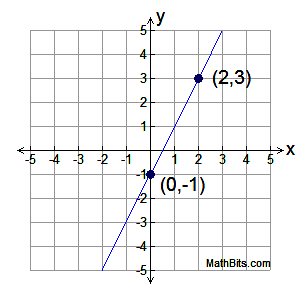Choose:
 m = 2 m = -2 m = 0.5 m = -0.5

5.
Which equation has a slope of -2 and a y-intercept
of 5?Choose:
 y = 2x + 5 y = 5x - 2 y = -2x + 5 y = 5x + 2

6.
Which chart belongs with the graph at the right?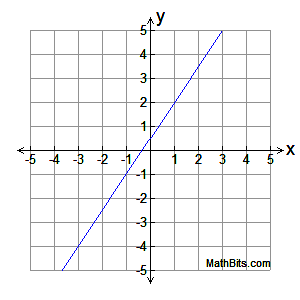Choose:

 x -3 -1 1 3 y -3 -1 2 4

 x -3 -1 1 3 y -4 -1 2 5

 x -3 -1 1 3 y -4 -1 1 5

 x -4 -1 2 5 y -3 -1 1 3

7.
Omar's garage is 10 feet above, and 100 feet back, from the road in front of his home.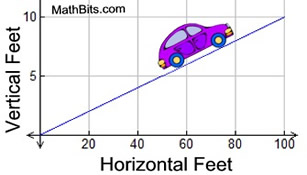a. What is the slope of his driveway?
Choose:
 m = 10 m = 5 m =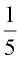m =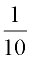b. Which equation could be used to model Omar's driveway?
Choose:
 y = 5x y = 10x y = 0.1x y = 0.2x
c. If Omar places a pole light on the edge of the driveway at a distance of 40 horizontal feet back from the road, how far above the road will the light be placed (in feet)?
Choose:

 2 feet 4 feet 4.5 feet 4.8 feet